# Workflow step examples

## Define environment settings and samples

A typical workflow starts with generating the expected working environment containing the proper directory structure, input files, and parameter settings. To simplify this task, one can load one of the existing NGS workflows templates provided by systemPipeRdata into the current working directory. The following does this for the rnaseq template. The name of the resulting workflow directory can be specified under the mydirname argument. The default NULL uses the name of the chosen workflow. An error is issued if a directory of the same name and path exists already. On Linux and OS X systems one can also create new workflow instances from the command-line of a terminal as shown here. To apply workflows to custom data, the user needs to modify the targets file and if necessary update the corresponding .cwl and .yml files. A collection of pre-generated .cwl and .yml files are provided in the param/cwl subdirectory of each workflow template. They are also viewable in the GitHub repository of systemPipeRdata (see here).

library(systemPipeR)
library(systemPipeRdata)
genWorkenvir(workflow = "rnaseq", mydirname = NULL)

##  "Generated rnaseq directory. Next run in rnaseq directory, the R code from *.Rmd template interactively. Alternatively, workflows can be exectued with a single command as instructed in the vignette."

setwd("rnaseq")


## Project initialization

To create a Workflow within systemPipeR, we can start by defining an empty container and checking the directory structure:

sal <- SPRproject()

## Creating directory '/home/lab/Desktop/spr/systemPipeR.github.io/content/en/sp/spr/rnaseq/.SPRproject'
## Creating file '/home/lab/Desktop/spr/systemPipeR.github.io/content/en/sp/spr/rnaseq/.SPRproject/SYSargsList.yml'


### Required packages and resources

The systemPipeR package needs to be loaded (H Backman and Girke 2016).

appendStep(sal) <- LineWise({
library(systemPipeR)


### Preprocessing with preprocessReads function

The function preprocessReads allows to apply predefined or custom read preprocessing functions to all FASTQ files referenced in a SYSargsList container, such as quality filtering or adaptor trimming routines. Internally, preprocessReads uses the FastqStreamer function from the ShortRead package to stream through large FASTQ files in a memory-efficient manner. The following example performs adaptor trimming with the trimLRPatterns function from the Biostrings package.

Here, we are appending this step at the SYSargsList object created previously. All the parameters are defined on the preprocessReads/preprocessReads-se.yml file.

targetspath <- system.file("extdata", "targets.txt", package = "systemPipeR")
appendStep(sal) <- SYSargsList(step_name = "preprocessing", targets = targetspath,
dir_path = system.file("extdata/cwl", package = "systemPipeR"), inputvars = c(FileName = "_FASTQ_PATH1_",
SampleName = "_SampleName_"), dependency = "load_SPR")


The paths to the resulting output FASTQ files can be checked as follows:

outfiles(sal)[]

## DataFrame with 18 rows and 1 column
##                 <character>
## M1A  ./results/M1A.fastq_..
## M1B  ./results/M1B.fastq_..
## A1A  ./results/A1A.fastq_..
## A1B  ./results/A1B.fastq_..
## V1A  ./results/V1A.fastq_..
## ...                     ...
## M12B ./results/M12B.fastq..
## A12A ./results/A12A.fastq..
## A12B ./results/A12B.fastq..
## V12A ./results/V12A.fastq..
## V12B ./results/V12B.fastq..


After the trimming step a new targets file is generated containing the paths to the trimmed FASTQ files. The new targets information can be used for the next workflow step instance, e.g. running the NGS alignments with the trimmed FASTQ files.

The following example shows how one can design a custom read preprocessing function using utilities provided by the ShortRead package, and then run it in batch mode with the ‘preprocessReads’ function. For here, it is possible to replace the function used on the preprocessing step and modify the sal object. First, we defined the function:

appendStep(sal) <- LineWise({
filterFct <- function(fq, cutoff = 20, Nexceptions = 0) {
qcount <- rowSums(as(quality(fq), "matrix") <= cutoff, na.rm = TRUE)
# Retains reads where Phred scores are >= cutoff with N exceptions
fq[qcount <= Nexceptions]
}
}, step_name = "custom_preprocessing_function", dependency = "preprocessing")


After, we can edit the input parameter:

yamlinput(sal, 2)$Fct yamlinput(sal, 2, "Fct") <- "'filterFct(fq, cutoff=20, Nexceptions=0)'" yamlinput(sal, 2)$Fct  ## check the new function


### Preprocessing with TrimGalore!

TrimGalore! is a wrapper tool to consistently apply quality and adapter trimming to fastq files, with some extra functionality for removing Reduced Representation Bisulfite-Seq (RRBS) libraries.

targetspath <- system.file("extdata", "targets.txt", package = "systemPipeR")
appendStep(sal) <- SYSargsList(step_name = "trimGalore", targets = targetspath, dir = TRUE,
wf_file = "trim_galore/trim_galore-se.cwl", input_file = "trim_galore/trim_galore-se.yml",
dir_path = system.file("extdata/cwl", package = "systemPipeR"), inputvars = c(FileName = "_FASTQ_PATH1_",
SampleName = "_SampleName_"), dependency = "load_SPR", run_step = "optional")


### Preprocessing with Trimmomatic

targetspath <- system.file("extdata", "targets.txt", package = "systemPipeR")
appendStep(sal) <- SYSargsList(step_name = "trimmomatic", targets = targetspath,
dir = TRUE, wf_file = "trimmomatic/trimmomatic-se.cwl", input_file = "trimmomatic/trimmomatic-se.yml",
dir_path = system.file("extdata/cwl", package = "systemPipeR"), inputvars = c(FileName = "_FASTQ_PATH1_",
SampleName = "_SampleName_"), dependency = "load_SPR", run_step = "optional")


## FASTQ quality report

The following seeFastq and seeFastqPlot functions generate and plot a series of useful quality statistics for a set of FASTQ files including per cycle quality box plots, base proportions, base-level quality trends, relative k-mer diversity, length and occurrence distribution of reads, number of reads above quality cutoffs and mean quality distribution.
The function seeFastq computes the quality statistics and stores the results in a relatively small list object that can be saved to disk with save() and reloaded with load() for later plotting. The argument klength specifies the k-mer length and batchsize the number of reads to a random sample from each FASTQ file.

appendStep(sal) <- LineWise({
files <- getColumn(sal, step = "preprocessing", "outfiles")  # get outfiles from preprocessing step
fqlist <- seeFastq(fastq = files, batchsize = 10000, klength = 8)
pdf("./results/fastqReport.pdf", height = 18, width = 4 * length(fqlist))
seeFastqPlot(fqlist)
dev.off()
}, step_name = "fastq_quality", dependency = "preprocessing")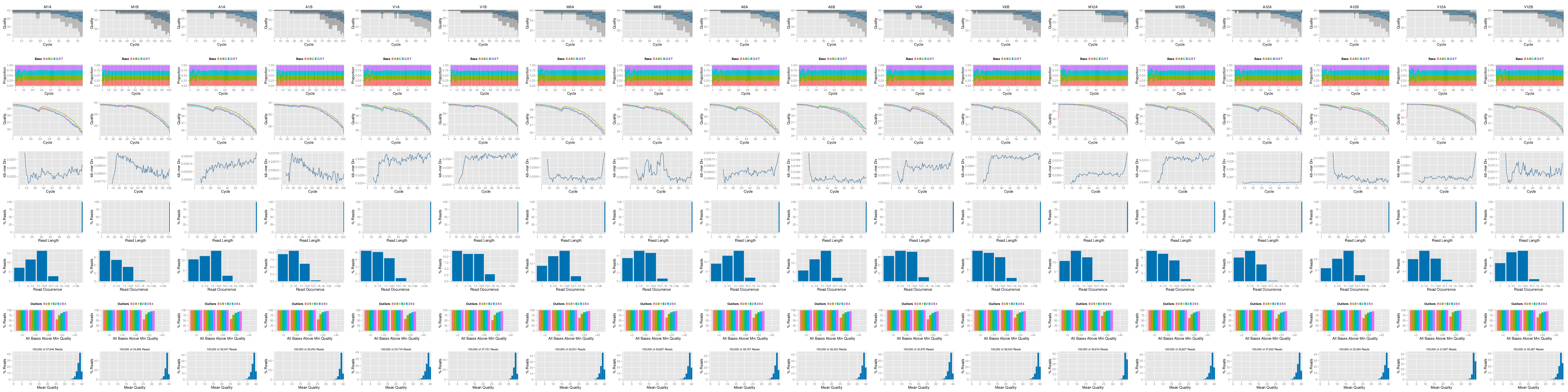Figure 1: FASTQ quality report

Parallelization of FASTQ quality report on a single machine with multiple cores.

appendStep(sal) <- LineWise({
files <- getColumn(sal, step = "preprocessing", "outfiles")  # get outfiles from preprocessing step
f <- function(x) seeFastq(fastq = files[x], batchsize = 1e+05, klength = 8)
fqlist <- bplapply(seq(along = files), f, BPPARAM = MulticoreParam(workers = 4))  ## Number of workers = 4
pdf("./results/fastqReport.pdf", height = 18, width = 4 * length(fqlist))
seeFastqPlot(unlist(fqlist, recursive = FALSE))
dev.off()
}, step_name = "fastq_quality", dependency = "preprocessing")


## NGS Alignment software

After quality control, the sequence reads can be aligned to a reference genome or transcriptome database. The following sessions present some NGS sequence alignment software. Select the most accurate aligner and determining the optimal parameter for your custom data set project.

For all the following examples, it is necessary to install the respective software and export the PATH accordingly. If it is available Environment Module in the system, you can load all the request software with moduleload(args) function.

### Alignment with HISAT2

The following steps will demonstrate how to use the short read aligner Hisat2 (Kim, Langmead, and Salzberg 2015) in both interactive job submissions and batch submissions to queuing systems of clusters using the systemPipeR's new CWL command-line interface.

• Build Hisat2 index.
appendStep(sal) <- SYSargsList(step_name = "hisat_index", targets = NULL, dir = FALSE,
wf_file = "hisat2/hisat2-index.cwl", input_file = "hisat2/hisat2-index.yml",
dir_path = system.file("extdata/cwl", package = "systemPipeR"), inputvars = NULL,
dependency = "preprocessing")


The parameter settings of the aligner are defined in the workflow_hisat2-se.cwl and workflow_hisat2-se.yml files. The following shows how to construct the corresponding SYSargsList object, and append to sal workflow.

• Alignment with HISAT2 and SAMtools

It possible to build an workflow with HISAT2 and SAMtools.

appendStep(sal) <- SYSargsList(step_name = "hisat_mapping", targets = "preprocessing",
dir = TRUE, wf_file = "workflow-hisat2/workflow_hisat2-se.cwl", input_file = "workflow-hisat2/workflow_hisat2-se.yml",
dir_path = system.file("extdata/cwl", package = "systemPipeR"), inputvars = c(preprocessReads_se = "_FASTQ_PATH1_",
SampleName = "_SampleName_"), dependency = c("hisat_index"))


### Alignment with Tophat2

The NGS reads of this project can also be aligned against the reference genome sequence using Bowtie2/TopHat2 (Kim et al. 2013; Langmead and Salzberg 2012).

• Build Bowtie2 index.
appendStep(sal) <- SYSargsList(step_name = "bowtie_index", targets = NULL, dir = FALSE,
wf_file = "bowtie2/bowtie2-index.cwl", input_file = "bowtie2/bowtie2-index.yml",
dir_path = system.file("extdata/cwl", package = "systemPipeR"), inputvars = NULL,
dependency = "preprocessing", run_step = "optional")


The parameter settings of the aligner are defined in the workflow_tophat2-mapping.cwl and tophat2-mapping-pe.yml files. The following shows how to construct the corresponding SYSargsList object, using the outfiles from the preprocessing step.

appendStep(sal) <- SYSargsList(step_name = "tophat2_mapping", targets = "preprocessing",
dir = TRUE, wf_file = "tophat2/workflow_tophat2-mapping-se.cwl", input_file = "tophat2/tophat2-mapping-se.yml",
dir_path = system.file("extdata/cwl", package = "systemPipeR"), inputvars = c(preprocessReads_se = "_FASTQ_PATH1_",
SampleName = "_SampleName_"), dependency = c("bowtie_index"), run_session = "compute",
run_step = "optional")


### Alignment with Bowtie2 (e.g. for miRNA profiling)

The following example runs Bowtie2 as a single process without submitting it to a cluster.

appendStep(sal) <- SYSargsList(step_name = "bowtie2_mapping", targets = "preprocessing",
dir = TRUE, wf_file = "bowtie2/workflow_bowtie2-mapping-se.cwl", input_file = "bowtie2/bowtie2-mapping-se.yml",
dir_path = system.file("extdata/cwl", package = "systemPipeR"), inputvars = c(preprocessReads_se = "_FASTQ_PATH1_",
SampleName = "_SampleName_"), dependency = c("bowtie_index"), run_session = "compute",
run_step = "optional")


### Alignment with BWA-MEM (e.g. for VAR-Seq)

The following example runs BWA-MEM as a single process without submitting it to a cluster.

• Build the index:
appendStep(sal) <- SYSargsList(step_name = "bwa_index", targets = NULL, dir = FALSE,
wf_file = "bwa/bwa-index.cwl", input_file = "bwa/bwa-index.yml", dir_path = system.file("extdata/cwl",
package = "systemPipeR"), inputvars = NULL, dependency = "preprocessing",
run_step = "optional")

• Prepare the alignment step:
appendStep(sal) <- SYSargsList(step_name = "bwa_mapping", targets = "preprocessing",
dir = TRUE, wf_file = "bwa/bwa-se.cwl", input_file = "bwa/bwa-se.yml", dir_path = system.file("extdata/cwl",
package = "systemPipeR"), inputvars = c(preprocessReads_se = "_FASTQ_PATH1_",
SampleName = "_SampleName_"), dependency = c("bwa_index"), run_session = "compute",
run_step = "optional")


### Alignment with Rsubread (e.g. for RNA-Seq)

The following example shows how one can use within the environment the R-based aligner , allowing running from R or command-line.

• Build the index:
appendStep(sal) <- SYSargsList(step_name = "rsubread_index", targets = NULL, dir = FALSE,
dir_path = system.file("extdata/cwl", package = "systemPipeR"), inputvars = NULL,
dependency = "preprocessing", run_step = "optional")

• Prepare the alignment step:
appendStep(sal) <- SYSargsList(step_name = "rsubread", targets = "preprocessing",
dir_path = system.file("extdata/cwl", package = "systemPipeR"), inputvars = c(preprocessReads_se = "_FASTQ_PATH1_",
SampleName = "_SampleName_"), dependency = c("rsubread_index"), run_session = "compute",
run_step = "optional")


### Alignment with gsnap (e.g. for VAR-Seq and RNA-Seq)

Another R-based short read aligner is gsnap from the gmapR package (Wu and Nacu 2010). The code sample below introduces how to run this aligner on multiple nodes of a compute cluster.

• Build the index:
appendStep(sal) <- SYSargsList(step_name = "gsnap_index", targets = NULL, dir = FALSE,
wf_file = "gsnap/gsnap-index.cwl", input_file = "gsnap/gsnap-index.yml", dir_path = system.file("extdata/cwl",
package = "systemPipeR"), inputvars = NULL, dependency = "preprocessing",
run_step = "optional")

• Prepare the alignment step:
appendStep(sal) <- SYSargsList(step_name = "gsnap", targets = "targetsPE.txt", dir = TRUE,
wf_file = "gsnap/gsnap-mapping-pe.cwl", input_file = "gsnap/gsnap-mapping-pe.yml",
dir_path = system.file("extdata/cwl", package = "systemPipeR"), inputvars = c(FileName1 = "_FASTQ_PATH1_",
FileName2 = "_FASTQ_PATH2_", SampleName = "_SampleName_"), dependency = c("gsnap_index"),
run_session = "compute", run_step = "optional")


The genome browser IGV supports reading of indexed/sorted BAM files via web URLs. This way it can be avoided to create unnecessary copies of these large files. To enable this approach, an HTML directory with Http access needs to be available in the user account (e.g. home/publichtml) of a system. If this is not the case then the BAM files need to be moved or copied to the system where IGV runs. In the following, htmldir defines the path to the HTML directory with http access where the symbolic links to the BAM files will be stored. The corresponding URLs will be written to a text file specified under the _urlfile_ argument.

appendStep(sal) <- LineWise({
symLink2bam(sysargs = stepsWF(sal)[], htmldir = c("~/.html/", "somedir/"),
urlbase = "http://myserver.edu/~username/", urlfile = "IGVurl.txt")
}, step_name = "igv", dependency = "hisat_mapping")


## Read counting for mRNA profiling experiments

Create txdb (needs to be done only once).

appendStep(sal) <- LineWise({
library(GenomicFeatures)
txdb <- makeTxDbFromGFF(file = "data/tair10.gff", format = "gff", dataSource = "TAIR",
organism = "Arabidopsis thaliana")
saveDb(txdb, file = "./data/tair10.sqlite")
}, step_name = "create_txdb", dependency = "hisat_mapping")


The following performs read counting with summarizeOverlaps in parallel mode with multiple cores.

appendStep(sal) <- LineWise({
library(BiocParallel)
eByg <- exonsBy(txdb, by = "gene")
outpaths <- getColumn(sal, step = "hisat_mapping", "outfiles", column = 2)
bfl <- BamFileList(outpaths, yieldSize = 50000, index = character())
multicoreParam <- MulticoreParam(workers = 4)
register(multicoreParam)
registered()
counteByg <- bplapply(bfl, function(x) summarizeOverlaps(eByg, x, mode = "Union",
ignore.strand = TRUE, inter.feature = TRUE, singleEnd = TRUE))
# Note: for strand-specific RNA-Seq set 'ignore.strand=FALSE' and for PE
# data set 'singleEnd=FALSE'
countDFeByg <- sapply(seq(along = counteByg), function(x) assays(counteByg[[x]])counts) rownames(countDFeByg) <- names(rowRanges(counteByg[])) colnames(countDFeByg) <- names(bfl) rpkmDFeByg <- apply(countDFeByg, 2, function(x) returnRPKM(counts = x, ranges = eByg)) write.table(countDFeByg, "results/countDFeByg.xls", col.names = NA, quote = FALSE, sep = "\t") write.table(rpkmDFeByg, "results/rpkmDFeByg.xls", col.names = NA, quote = FALSE, sep = "\t") }, step_name = "read_counting", dependency = "create_txdb")  Please note, in addition to read counts this step generates RPKM normalized expression values. For most statistical differential expression or abundance analysis methods, such as edgeR or DESeq2, the raw count values should be used as input. The usage of RPKM values should be restricted to specialty applications required by some users, e.g. manually comparing the expression levels of different genes or features. #### Read and alignment count stats Generate a table of read and alignment counts for all samples. appendStep(sal) <- LineWise({ read_statsDF <- alignStats(args) write.table(read_statsDF, "results/alignStats.xls", row.names = FALSE, quote = FALSE, sep = "\t") }, step_name = "align_stats", dependency = "hisat_mapping")  The following shows the first four lines of the sample alignment stats file provided by the systemPipeR package. For simplicity the number of PE reads is multiplied here by 2 to approximate proper alignment frequencies where each read in a pair is counted. read.table(system.file("extdata", "alignStats.xls", package = "systemPipeR"), header = TRUE)[1:4, ]  ## FileName Nreads2x Nalign Perc_Aligned Nalign_Primary Perc_Aligned_Primary ## 1 M1A 192918 177961 92.24697 177961 92.24697 ## 2 M1B 197484 159378 80.70426 159378 80.70426 ## 3 A1A 189870 176055 92.72397 176055 92.72397 ## 4 A1B 188854 147768 78.24457 147768 78.24457  ## Read counting for miRNA profiling experiments Download miRNA genes from miRBase. appendStep(sal) <- LineWise({ system("wget https://www.mirbase.org/ftp/CURRENT/genomes/ath.gff3 -P ./data/") gff <- rtracklayer::import.gff("./data/ath.gff3") gff <- split(gff, elementMetadata(gff)ID)
bams <- getColumn(sal, step = "bowtie2_mapping", "outfiles", column = 2)
bfl <- BamFileList(bams, yieldSize = 50000, index = character())
countDFmiR <- summarizeOverlaps(gff, bfl, mode = "Union", ignore.strand = FALSE,
inter.feature = FALSE)
countDFmiR <- assays(countDFmiR)$counts # Note: inter.feature=FALSE important since pre and mature miRNA ranges # overlap rpkmDFmiR <- apply(countDFmiR, 2, function(x) returnRPKM(counts = x, ranges = gff)) write.table(assays(countDFmiR)$counts, "results/countDFmiR.xls", col.names = NA,
quote = FALSE, sep = "\t")
write.table(rpkmDFmiR, "results/rpkmDFmiR.xls", col.names = NA, quote = FALSE,
sep = "\t")
}, step_name = "read_counting_mirna", dependency = "bowtie2_mapping")


## Correlation analysis of samples

The following computes the sample-wise Spearman correlation coefficients from the rlog (regularized-logarithm) transformed expression values generated with the DESeq2 package. After transformation to a distance matrix, hierarchical clustering is performed with the hclust function and the result is plotted as a dendrogram (sample_tree.pdf).

appendStep(sal) <- LineWise({
library(DESeq2, warn.conflicts = FALSE, quietly = TRUE)
library(ape, warn.conflicts = FALSE)
countDFpath <- system.file("extdata", "countDFeByg.xls", package = "systemPipeR")
colData <- data.frame(row.names = targetsWF(sal)[]$SampleName, condition = targetsWF(sal)[]$Factor)
dds <- DESeqDataSetFromMatrix(countData = countDF, colData = colData, design = ~condition)
d <- cor(assay(rlog(dds)), method = "spearman")
hc <- hclust(dist(1 - d))
plot.phylo(as.phylo(hc), type = "p", edge.col = 4, edge.width = 3, show.node.label = TRUE,
no.margin = TRUE)
}, step_name = "sample_tree_rlog", dependency = "read_counting")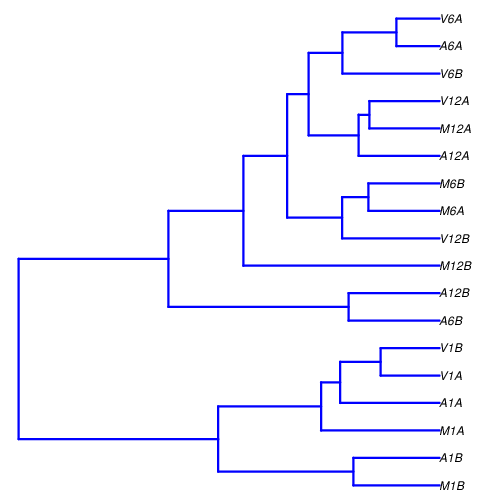Figure 2: Correlation dendrogram of samples for rlog values.

## DEG analysis with edgeR

The following *run_edgeR* function is a convenience wrapper for identifying differentially expressed genes (DEGs) in batch mode with *edgeR’s GML method (Robinson, McCarthy, and Smyth 2010) for any number of pairwise sample comparisons specified under the cmp* argument. Users are strongly encouraged to consult the edgeR vignette for more detailed information on this topic and how to properly run edgeR on data sets with more complex experimental designs.

appendStep(sal) <- LineWise({
targetspath <- system.file("extdata", "targets.txt", package = "systemPipeR")
targets <- read.delim(targetspath, comment = "#")
cmp <- readComp(file = targetspath, format = "matrix", delim = "-")
countDFeBygpath <- system.file("extdata", "countDFeByg.xls", package = "systemPipeR")
countDFeByg <- read.delim(countDFeBygpath, row.names = 1)
edgeDF <- run_edgeR(countDF = countDFeByg, targets = targets, cmp = cmp[],
independent = FALSE, mdsplot = "")
DEG_list <- filterDEGs(degDF = edgeDF, filter = c(Fold = 2, FDR = 10))
}, step_name = "edger", dependency = "read_counting")


Filter and plot DEG results for up and down-regulated genes. Because of the small size of the toy data set used by this vignette, the FDR value has been set to a relatively high threshold (here 10%). More commonly used FDR cutoffs are 1% or 5%. The definition of ‘up’ and ‘down’ is given in the corresponding help file. To open it, type ?filterDEGs in the R console.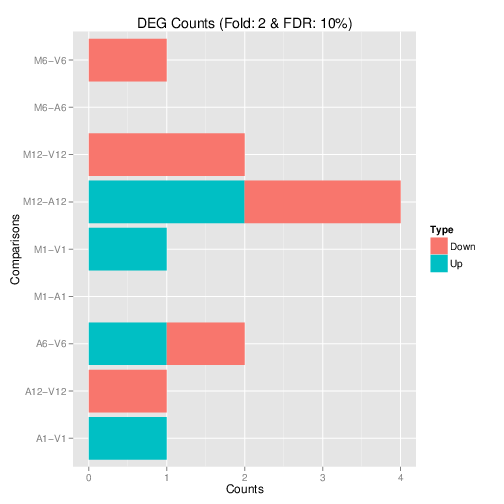Figure 3: Up and down regulated DEGs identified by edgeR.

## DEG analysis with DESeq2

The following *run_DESeq2* function is a convenience wrapper for identifying DEGs in batch mode with DESeq2* (Love, Huber, and Anders 2014) for any number of pairwise sample comparisons specified under the cmp* argument. Users are strongly encouraged to consult the DESeq2 vignette for more detailed information on this topic and how to properly run DESeq2 on data sets with more complex experimental designs.

appendStep(sal) <- LineWise({
degseqDF <- run_DESeq2(countDF = countDFeByg, targets = targets, cmp = cmp[],
independent = FALSE)
DEG_list2 <- filterDEGs(degDF = degseqDF, filter = c(Fold = 2, FDR = 10))
}, step_name = "deseq2", dependency = "read_counting")


## Venn Diagrams

The function overLapper can compute Venn intersects for large numbers of sample sets (up to 20 or more) and vennPlot can plot 2-5 way Venn diagrams. A useful feature is the possibility to combine the counts from several Venn comparisons with the same number of sample sets in a single Venn diagram (here for 4 up and down DEG sets).

appendStep(sal) <- LineWise({
vennsetup <- overLapper(DEG_list$Up[6:9], type = "vennsets") vennsetdown <- overLapper(DEG_list$Down[6:9], type = "vennsets")
vennPlot(list(vennsetup, vennsetdown), mymain = "", mysub = "", colmode = 2,
ccol = c("blue", "red"))
}, step_name = "vennplot", dependency = "edger")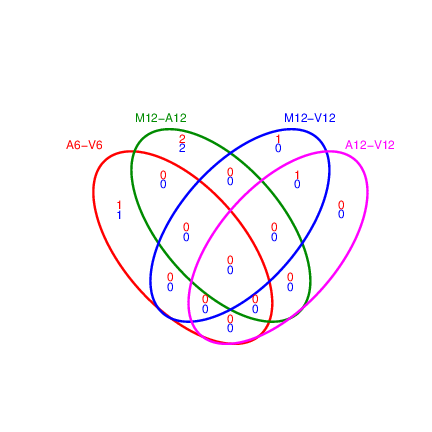Figure 4: Venn Diagram for 4 Up and Down DEG Sets.

## GO term enrichment analysis of DEGs

### Obtain gene-to-GO mappings

The following shows how to obtain gene-to-GO mappings from biomaRt (here for A. thaliana) and how to organize them for the downstream GO term enrichment analysis. Alternatively, the gene-to-GO mappings can be obtained for many organisms from Bioconductor’s *.db genome annotation packages or GO annotation files provided by various genome databases. For each annotation, this relatively slow preprocessing step needs to be performed only once. Subsequently, the preprocessed data can be loaded with the load function as shown in the next subsection.

appendStep(sal) <- LineWise({
library("biomaRt")
listMarts()  # To choose BioMart database
listMarts(host = "plants.ensembl.org")
m <- useMart("plants_mart", host = "https://plants.ensembl.org")
listDatasets(m)
m <- useMart("plants_mart", dataset = "athaliana_eg_gene", host = "https://plants.ensembl.org")
go <- getBM(attributes = c("go_id", "tair_locus", "namespace_1003"), mart = m)
go <- go[go[, 3] != "", ]
go[, 3] <- as.character(go[, 3])
go[go[, 3] == "molecular_function", 3] <- "F"
go[go[, 3] == "biological_process", 3] <- "P"
go[go[, 3] == "cellular_component", 3] <- "C"
go[1:4, ]
dir.create("./data/GO")
write.table(go, "data/GO/GOannotationsBiomart_mod.txt", quote = FALSE, row.names = FALSE,
col.names = FALSE, sep = "\t")
catdb <- makeCATdb(myfile = "data/GO/GOannotationsBiomart_mod.txt", lib = NULL,
org = "", colno = c(1, 2, 3), idconv = NULL)
save(catdb, file = "data/GO/catdb.RData")
}, step_name = "get_go_biomart", dependency = "edger")


### Batch GO term enrichment analysis

Apply the enrichment analysis to the DEG sets obtained in the above differential expression analysis. Note, in the following example the FDR filter is set here to an unreasonably high value, simply because of the small size of the toy data set used in this vignette. Batch enrichment analysis of many gene sets is performed with the GOCluster_Report function. When method="all", it returns all GO terms passing the p-value cutoff specified under the cutoff arguments. When method="slim", it returns only the GO terms specified under the myslimv argument. The given example shows how one can obtain such a GO slim vector from BioMart for a specific organism.

appendStep(sal) <- LineWise({
DEG_list <- filterDEGs(degDF = edgeDF, filter = c(Fold = 2, FDR = 50), plot = FALSE)
up_down <- DEG_list$UporDown names(up_down) <- paste(names(up_down), "_up_down", sep = "") up <- DEG_list$Up
names(up) <- paste(names(up), "_up", sep = "")
down <- DEG_list$Down names(down) <- paste(names(down), "_down", sep = "") DEGlist <- c(up_down, up, down) DEGlist <- DEGlist[sapply(DEGlist, length) > 0] BatchResult <- GOCluster_Report(catdb = catdb, setlist = DEGlist, method = "all", id_type = "gene", CLSZ = 2, cutoff = 0.9, gocats = c("MF", "BP", "CC"), recordSpecGO = NULL) library("biomaRt") m <- useMart("plants_mart", dataset = "athaliana_eg_gene", host = "https://plants.ensembl.org") goslimvec <- as.character(getBM(attributes = c("goslim_goa_accession"), mart = m)[, 1]) BatchResultslim <- GOCluster_Report(catdb = catdb, setlist = DEGlist, method = "slim", id_type = "gene", myslimv = goslimvec, CLSZ = 10, cutoff = 0.01, gocats = c("MF", "BP", "CC"), recordSpecGO = NULL) gos <- BatchResultslim[grep("M6-V6_up_down", BatchResultslim$CLID), ]
gos <- BatchResultslim
pdf("GOslimbarplotMF.pdf", height = 8, width = 10)
goBarplot(gos, gocat = "MF")
dev.off()
goBarplot(gos, gocat = "BP")
goBarplot(gos, gocat = "CC")
}, step_name = "go_enrichment", dependency = "get_go_biomart")


### Plot batch GO term results

The data.frame generated by GOCluster_Report can be plotted with the goBarplot function. Because of the variable size of the sample sets, it may not always be desirable to show the results from different DEG sets in the same bar plot. Plotting single sample sets is achieved by subsetting the input data frame as shown in the first line of the following example.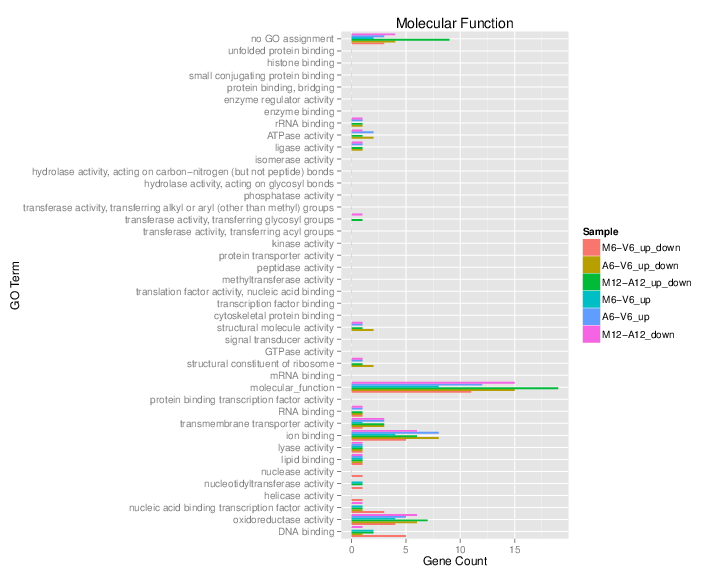Figure 5: GO Slim Barplot for MF Ontology.

## Clustering and heat maps

The following example performs hierarchical clustering on the rlog transformed expression matrix subsetted by the DEGs identified in the above differential expression analysis. It uses a Pearson correlation-based distance measure and complete linkage for cluster join.

appendStep(sal) <- LineWise({
library(pheatmap)
geneids <- unique(as.character(unlist(DEG_list[])))
y <- assay(rlog(dds))[geneids, ]
pdf("heatmap1.pdf")
pheatmap(y, scale = "row", clustering_distance_rows = "correlation", clustering_distance_cols = "correlation")
dev.off()
}, step_name = "hierarchical_clustering", dependency = c("sample_tree_rlog", "edger"))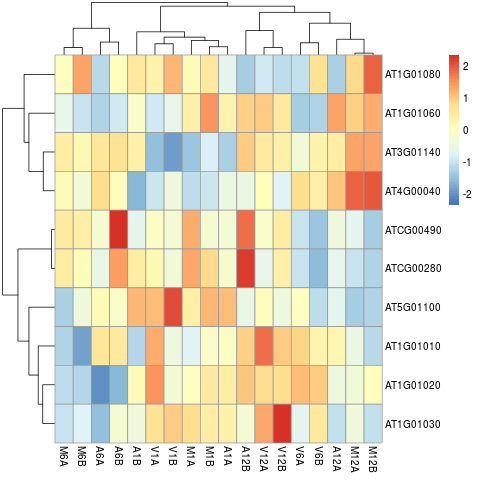Figure 7: Heat map with hierarchical clustering dendrograms of DEGs.

## Visualize workflow

systemPipeR workflows instances can be visualized with the plotWF function.

This function will make a plot of selected workflow instance and the following information is displayed on the plot:

• Workflow structure (dependency graphs between different steps);
• Workflow step status, e.g. Success, Error, Pending, Warnings;
• Sample status and statistics;
• Workflow timing: running duration time.

If no argument is provided, the basic plot will automatically detect width, height, layout, plot method, branches, etc.

plotWF(sal, show_legend = TRUE, width = "80%")


## Running the workflow

For running the workflow, runWF function will execute all the command lines store in the workflow container.

sal <- runWF(sal)


#### Interactive job submissions in a single machine

To simplify the short read alignment execution for the user, the command-line can be run with the runCommandline function. The execution will be on a single machine without submitting to a queuing system of a computer cluster. This way, the input FASTQ files will be processed sequentially. By default runCommandline auto detects SAM file outputs and converts them to sorted and indexed BAM files, using internally the Rsamtools package (Morgan et al. 2019). Besides, runCommandline allows the user to create a dedicated results folder for each workflow and a sub-folder for each sample defined in the targets file. This includes all the output and log files for each step. When these options are used, the output location will be updated by default and can be assigned to the same object.

If available, multiple CPU cores can be used for processing each file. The number of CPU cores (here 4) to use for each process is defined in the *.yml file. With yamlinput(args)['thread'] one can return this value from the SYSargs2 object.

#### Parallelization on clusters

Alternatively, the computation can be greatly accelerated by processing many files in parallel using several compute nodes of a cluster, where a scheduling/queuing system is used for load balancing. For this the clusterRun function submits the computing requests to the scheduler using the run specifications defined by runCommandline.

To avoid over-subscription of CPU cores on the compute nodes, the value from yamlinput(args)['thread'] is passed on to the submission command, here ncpus in the resources list object. The number of independent parallel cluster processes is defined under the Njobs argument. The following example will run 18 processes in parallel using for each 4 CPU cores. If the resources available on a cluster allow running all 18 processes at the same time then the shown sample submission will utilize in total 72 CPU cores. Note, clusterRun can be used with most queueing systems as it is based on utilities from the batchtools package which supports the use of template files (*.tmpl) for defining the run parameters of different schedulers. To run the following code, one needs to have both a conf file (see .batchtools.conf.R samples here) and a template file (see *.tmpl samples here) for the queueing available on a system. The following example uses the sample conf and template files for the Slurm scheduler provided by this package.

## Access the Previous Version

Please find here the previous version.

H Backman, Tyler W, and Thomas Girke. 2016. “systemPipeR: NGS workflow and report generation environment.” BMC Bioinformatics 17 (1): 388. https://doi.org/10.1186/s12859-016-1241-0.

Kim, Daehwan, Ben Langmead, and Steven L Salzberg. 2015. “HISAT: A Fast Spliced Aligner with Low Memory Requirements.” Nat. Methods 12 (4): 357–60.

Kim, Daehwan, Geo Pertea, Cole Trapnell, Harold Pimentel, Ryan Kelley, and Steven L Salzberg. 2013. “TopHat2: Accurate Alignment of Transcriptomes in the Presence of Insertions, Deletions and Gene Fusions.” Genome Biol. 14 (4): R36. https://doi.org/10.1186/gb-2013-14-4-r36.

Langmead, Ben, and Steven L Salzberg. 2012. “Fast Gapped-Read Alignment with Bowtie 2.” Nat. Methods 9 (4): 357–59. https://doi.org/10.1038/nmeth.1923.

Love, Michael, Wolfgang Huber, and Simon Anders. 2014. “Moderated Estimation of Fold Change and Dispersion for RNA-seq Data with DESeq2.” Genome Biol. 15 (12): 550. https://doi.org/10.1186/s13059-014-0550-8.

Morgan, Martin, Hervé Pagès, Valerie Obenchain, and Nathaniel Hayden. 2019. Rsamtools: Binary Alignment (BAM), FASTA, Variant Call (BCF), and Tabix File Import. http://bioconductor.org/packages/Rsamtools.

Robinson, M D, D J McCarthy, and G K Smyth. 2010. “edgeR: A Bioconductor Package for Differential Expression Analysis of Digital Gene Expression Data.” Bioinformatics 26 (1): 139–40. https://doi.org/10.1093/bioinformatics/btp616.

Wu, T D, and S Nacu. 2010. “Fast and SNP-tolerant Detection of Complex Variants and Splicing in Short Reads.” Bioinformatics 26 (7): 873–81. https://doi.org/10.1093/bioinformatics/btq057.